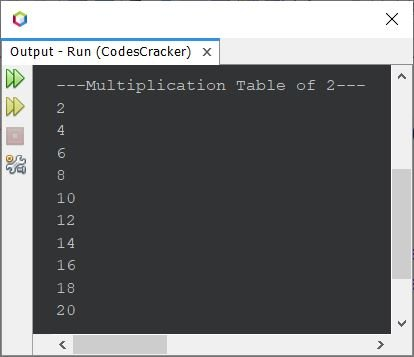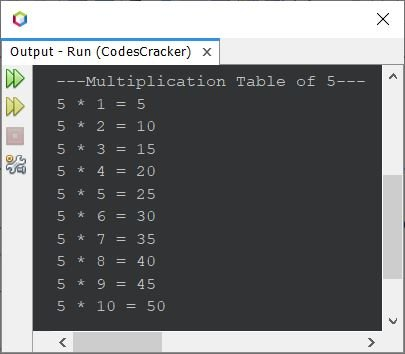# Java Program to Print Table of a Number

This article covers multiple programs in Java that find and prints the multiplication table of a number. List of programs covered in this article are:

• Print multiplication table of 2
• Print multiplication table using while loop
• Print multiplication table of any given number

## Print Multiplication Table of 2 in Java

The question is, write a Java program to print multiplication table of 2. The program given below is its answer:

```public class CodesCracker
{
public static void main(String[] args)
{
int num=2, i;
System.out.println("\n---Multiplication Table of 2---");

for(i=1; i<=10; i++)
System.out.println(num*i);
}
}```

The snapshot given below shows the sample output produced by above program, on printing the multiplication table of 2The above program can also be written in this way, to produce interactive output, than previous one:

```public class CodesCracker
{
public static void main(String[] args)
{
int num=2, i;
System.out.println("\n---Multiplication Table of 2---");
for(i=1; i<=10; i++)
System.out.println(num+ " * " +i+ " = " +(num*i));
}
}```

Now the output will be:

```---Multiplication Table of 2---
2 * 1 = 2
2 * 2 = 4
2 * 3 = 6
2 * 4 = 8
2 * 5 = 10
2 * 6 = 12
2 * 7 = 14
2 * 8 = 16
2 * 9 = 18
2 * 10 = 20```

## Print Multiplication Table using while Loop in Java

The previous program can also be created using while loop, as shown in the program given below:

```public class CodesCracker
{
public static void main(String[] args)
{
int num=2, i=1;
System.out.println("\n---Multiplication Table of 2---");
while(i<=10)
{
System.out.println(num+ " * " +i+ " = " +(num*i));
i++;
}
}
}```

This program produces same output as of previous one.

## Print Multiplication Table of any Given Number in Java

This program allows user to enter the number, of which, he/she wants to print the multiplication table.

```import java.util.Scanner;

public class CodesCracker
{
public static void main(String[] args)
{
Scanner scan = new Scanner(System.in);

System.out.print("Enter the Number: ");
int num = scan.nextInt();

System.out.println("\n---Multiplication Table of " +num+ "---");
for(int i=1; i<=10; i++)
System.out.println(num+ " * " +i+ " = " +(num*i));
}
}```

The sample run of above program with user input 5 as number to print the multiplication table of 5 is shown in the snapshot given below:#### Same Program in Other Languages

Java Online Test

« Previous Program Next Program »